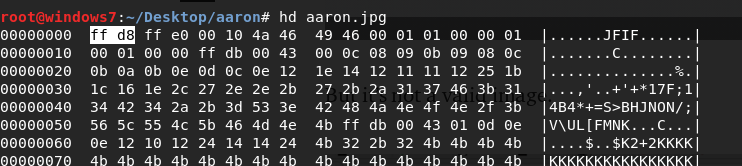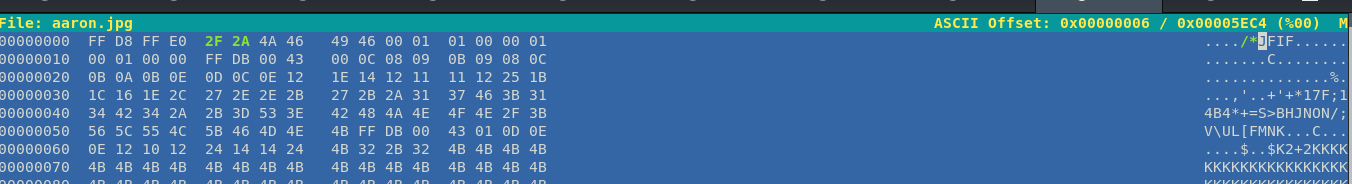jpg文件以FF D8开头，以FF D9结束，中间包含以FF XX(X表示十六进制数)开头的不同块。```import binascii

ff = open('test.jpg', 'rb')

ff.close()

jpgHex = binascii.b2a_hex(f).decode('utf-8')

c = jpgHex[0 : 8] + '2f2a' + jpgHex[12:40]

d = '00' * (12058 - len(e) // 2)

f = jpgHex[40 :]

g = c + d + e + f + '2a2f'

new = binascii.a2b_hex(g)

ff = open('new.jpg', 'wb+')

ff.write(new)

ff.close()

```

```from PIL import Image

im = Image.new("RGB", (1, 1))

im.putpixel((0, 0), (255, 255, 255))

im.save('flag.jpg')

```